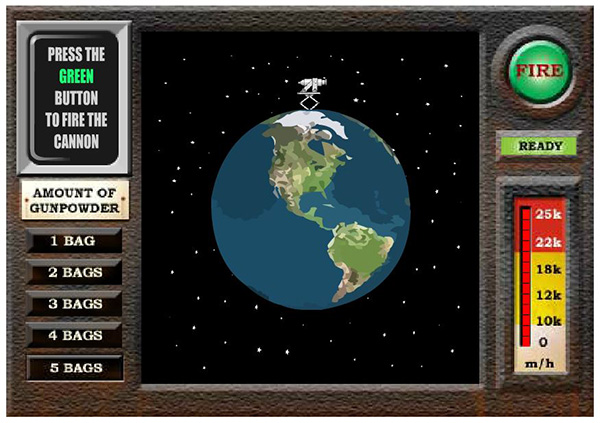Circular orbitsSatellites are held in orbit by the gravitational force of the planet on the satellite. We will investigate the fundamental concepts regarding a satellite in orbit that is moving without any rocket power.An orbiting object is basically a body in free-fall. It is moving fast enough that as it falls, the surface of the planet curves away from it so that the object never lands. This concept is illustrated in a thought experiment called Newton's cannon.

The speed of an orbiting satellite depends on its altitude. A satellite with an elliptical orbit moves faster when it is close to the planet than when it is farther away. For circular orbits, a satellite in a low orbit needs to move faster than a satellite in a higher orbit.

Geosynchronous orbits

In general, a satellite orbiting a planet orbits around the center of mass the planet. It can orbit in any circle, as long as the center of mass of the planet is at the center of the circle. A satellite can have an orbit that carries it over the north and south pole, for example.

A satellite that has the same rotation period of the Earth is called a "geosynchronous" satellite. To maintain a geosynchronous orbit, a satellite needs to be at an altitude of about 3.58x107 m.

A satellite can have an orbit that lies directly over the equator. If a satellite has an orbit lying directly above the equator and the same period as the rotational period of Earth, it remains over a single point on the surface of Earth. Such a satellite is called a "geostationary" satellite.

Example problems:

1. What is the speed of a geostationary satellite?

2. What is the acceleration due to gravity at the altitude of a geostationary satellite?

3. What is the weight of a geostationary satellite?